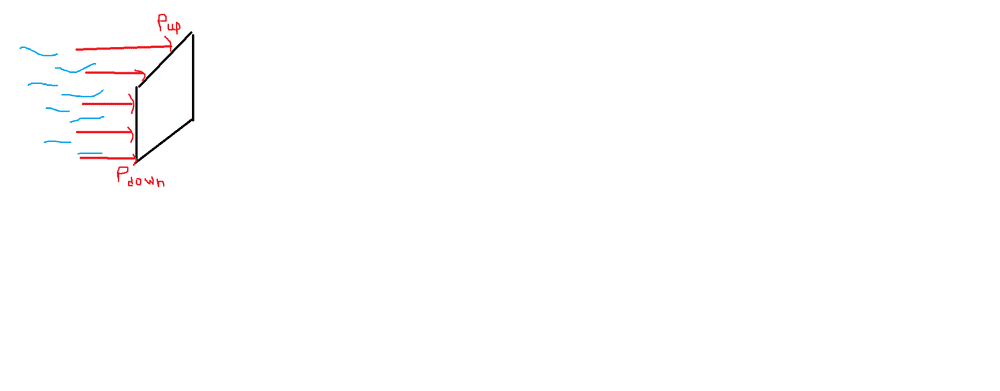# Lateral pressure of a liquid exerted on a plunger

A13235378
Homework Statement:
Consider a liquid exerting a force on a plunger. Find that force

I saw somewhere that I don't remember anymore that you can use medium force, specifically medium pressure:

(P up + P down) / 2

I also saw the following expression:

dF = (P + ugh) dS that represents the force exerted by each minuscule piece.

I was wondering if those two expressions are right and how can I start from the second and get to the first. In short, I wanted to better understand the concept of average quantities. In speed for example, I can use ( Vinitial+ Vfinal) / 2 to find the average speed, that is, when I can do a simple arithmetic medium.
Relevant Equations:
P2 = P1 + ugh , u = Especific mass.•To complement what @haruspex said, orient the rectangular plate, of width ##w## and height ##h##, in the ##x##-##y## plane, and consider the fluid to occupy ##z < 0##. The pressure force will be$$F_z = \int_0^w \int_0^h P_0 - \rho g y \, dy \, dx = \int_0^w P_0 h - \frac{1}{2}\rho g h^2 \, dx = P_0 h w - \frac{1}{2}\rho g h^2 w$$The pressures you labelled would be ##P_{down} = P_0## and ##P_{up} = P_0 - \rho g h##. So looking at the average pressure, the force can be written as $$\frac{P_{up} + P_{down}}{2} homework = P_0 homework - \frac{1}{2} \rho g h^2 w$$which is the same as we obtained with the general expression. This only occurs here because the pressure variation is linear with depth and because the width of the lamina is constant. In general it will not hold, in just the same way that something like SUVAT will not hold unless certain criteria are met!